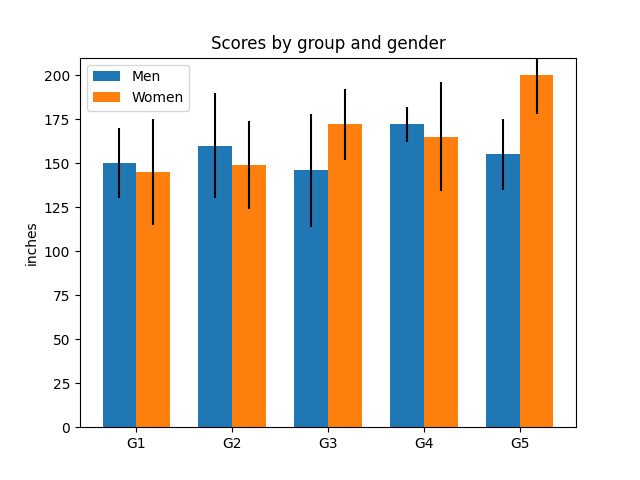# Group barchart with units#

This is the same example as the barchart in centimeters.

This example requires basic_units.pyimport numpy as np
from basic_units import cm, inch
import matplotlib.pyplot as plt

N = 5
men_means = [150*cm, 160*cm, 146*cm, 172*cm, 155*cm]
men_std = [20*cm, 30*cm, 32*cm, 10*cm, 20*cm]

fig, ax = plt.subplots()

ind = np.arange(N)    # the x locations for the groups
width = 0.35         # the width of the bars
ax.bar(ind, men_means, width, bottom=0*cm, yerr=men_std, label='Men')

women_means = (145*cm, 149*cm, 172*cm, 165*cm, 200*cm)
women_std = (30*cm, 25*cm, 20*cm, 31*cm, 22*cm)
ax.bar(ind + width, women_means, width, bottom=0*cm, yerr=women_std,
label='Women')

ax.set_title('Scores by group and gender')
ax.set_xticks(ind + width / 2, labels=['G1', 'G2', 'G3', 'G4', 'G5'])

ax.legend()
ax.yaxis.set_units(inch)
ax.autoscale_view()

plt.show()


Gallery generated by Sphinx-Gallery